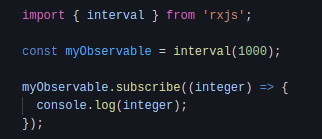# Create an RxJS Observable with IntervalAs reported in the documentation, “Most commonly, observables are created using creation functions, like of, from, interval, etc”.

Following this recommendation, we will use the RxJS `interval` function to create an Observable by using built-in creation functions. Once again, it doesn't matter if you use a framework like Angular or a library like React. RxJS is independent.

### Creating an Observable

Once again, the process to create an Observable is fairly straightforward.

First of all, we need to import `interval` from RxJS.

The `interval` built-in function is simply a function that "Creates an Observable that emits sequential numbers every specified interval of time", rxjs.dev.

In other words, thanks to `interval` we will get a stream (Observable) of ascending numbers. The Observable will emit integers, "with a constant interval of time of your choosing between those emissions".

In the following piece of code, we are creating an Observable that emits a sequential number every second (1000 ms).

``````import { interval } from "rxjs";
const myObservable = interval(1000);
``````

As it is, nothing will happen because we need to subscribe to an Observable to consume its values.

### Subscribing to the Observable

The first step required us to create an Observable. Now, we want to log the values that are coming from this Observable to the console.

``````import { interval } from "rxjs";
const myObservable = interval(1000);
myObservable.subscribe((integer) => {
console.log(integer);
});
``````

It is as simple as that. myObservable is now emitting a stream of integers every second and you can see that in the console.

Even though we are not using any of the Observables notifications (`next`, `error` and `complete`), the Observable is implicitly using `next` to send data.

Create an Observable using RxJS interval:A built-in Observable like `interval` has several advantages over custom Observables.
On the other side, some built-in creation functions like `of` and `from` will complete on their own.